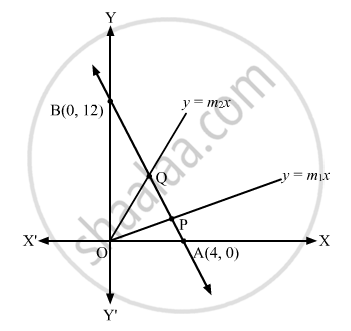Advertisement Remove all ads

# Find the Equations to the Straight Lines Which Go Through the Origin and Trisect the Portion of the Straight Line 3 X + Y = 12 Which is Intercepted Between the Axes of Coordinates. - Mathematics

Answer in Brief

Find the equations to the straight lines which go through the origin and trisect the portion of the straight line 3 x + y = 12 which is intercepted between the axes of coordinates.

Advertisement Remove all ads

#### Solution

Let the line 3x + y = 12 intersect the x-axis and the y-axis at A and B, respectively.
At x = 0
0 + y = 12

$\Rightarrow$ y = 12

At y = 0
3x + 0 = 12

$\Rightarrow$ x = 4

$\therefore A \equiv \left( 4, 0 \right) \text{and } B \equiv \left( 0, 12 \right)$

Let

$y = m_1 x \text { and } y = m_2 x$ be the lines that pass through the origin and trisect the line 3x + y = 12 at P and Q.
∴ AP = PQ = QB
Let us find the coordinates of P and Q.

$P \equiv \left( \frac{2 \times 4 + 1 \times 0}{2 + 1}, \frac{2 \times 0 + 1 \times 12}{2 + 1} \right) \equiv \left( \frac{8}{3}, 4 \right)$

$Q \equiv \left( \frac{1 \times 4 + 2 \times 0}{2 + 1}, \frac{1 \times 0 + 2 \times 12}{2 + 1} \right) \equiv \left( \frac{4}{3}, 8 \right)$

Clearly, P and Q lie on $y = m_1 x \text { and } y = m_2 x$ ,respectively.

$\therefore 4 = m_1 \times \frac{8}{3} \text { and }8 = m_2 \times \frac{4}{3}$

$\Rightarrow m_1 = \frac{3}{2} \text { and} m_2 = 6$

Hence, the required lines are

$y = \frac{3}{2}x \Rightarrow 2y = 3x \text { and } y = 6x$Concept: Straight Lines - Equation of Family of Lines Passing Through the Point of Intersection of Two Lines
Is there an error in this question or solution?
Advertisement Remove all ads

#### APPEARS IN

RD Sharma Class 11 Mathematics Textbook
Chapter 23 The straight lines
Exercise 23.5 | Q 14 | Page 35
Advertisement Remove all ads
Advertisement Remove all ads
Share
Notifications

View all notifications

Forgot password?